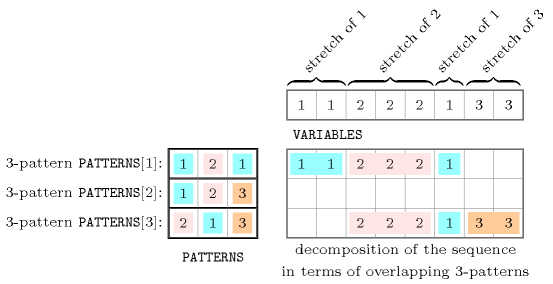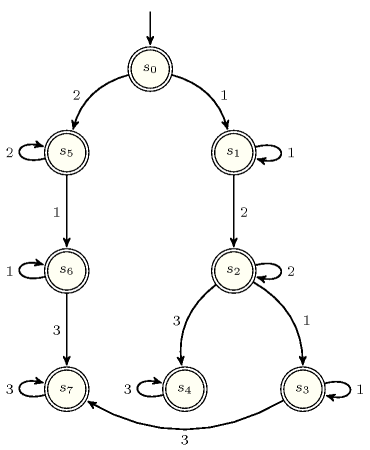## 5.317. pattern

Origin
Constraint

$\mathrm{𝚙𝚊𝚝𝚝𝚎𝚛𝚗}\left(\mathrm{𝚅𝙰𝚁𝙸𝙰𝙱𝙻𝙴𝚂},\mathrm{𝙿𝙰𝚃𝚃𝙴𝚁𝙽𝚂}\right)$

Type
 $\mathrm{𝙿𝙰𝚃𝚃𝙴𝚁𝙽}$ $\mathrm{𝚌𝚘𝚕𝚕𝚎𝚌𝚝𝚒𝚘𝚗}\left(\mathrm{𝚟𝚊𝚛}-\mathrm{𝚒𝚗𝚝}\right)$
Arguments
 $\mathrm{𝚅𝙰𝚁𝙸𝙰𝙱𝙻𝙴𝚂}$ $\mathrm{𝚌𝚘𝚕𝚕𝚎𝚌𝚝𝚒𝚘𝚗}\left(\mathrm{𝚟𝚊𝚛}-\mathrm{𝚍𝚟𝚊𝚛}\right)$ $\mathrm{𝙿𝙰𝚃𝚃𝙴𝚁𝙽𝚂}$ $\mathrm{𝚌𝚘𝚕𝚕𝚎𝚌𝚝𝚒𝚘𝚗}\left(\mathrm{𝚙𝚊𝚝}-\mathrm{𝙿𝙰𝚃𝚃𝙴𝚁𝙽}\right)$
Restrictions
 $\mathrm{𝚛𝚎𝚚𝚞𝚒𝚛𝚎𝚍}$$\left(\mathrm{𝙿𝙰𝚃𝚃𝙴𝚁𝙽},\mathrm{𝚟𝚊𝚛}\right)$ $\mathrm{𝙿𝙰𝚃𝚃𝙴𝚁𝙽}.\mathrm{𝚟𝚊𝚛}\ge 0$ $\mathrm{𝚌𝚑𝚊𝚗𝚐𝚎}$$\left(0,\mathrm{𝙿𝙰𝚃𝚃𝙴𝚁𝙽},=\right)$ $|\mathrm{𝙿𝙰𝚃𝚃𝙴𝚁𝙽}|>1$ $\mathrm{𝚛𝚎𝚚𝚞𝚒𝚛𝚎𝚍}$$\left(\mathrm{𝚅𝙰𝚁𝙸𝙰𝙱𝙻𝙴𝚂},\mathrm{𝚟𝚊𝚛}\right)$ $\mathrm{𝚛𝚎𝚚𝚞𝚒𝚛𝚎𝚍}$$\left(\mathrm{𝙿𝙰𝚃𝚃𝙴𝚁𝙽𝚂},\mathrm{𝚙𝚊𝚝}\right)$ $|\mathrm{𝙿𝙰𝚃𝚃𝙴𝚁𝙽𝚂}|>0$ $\mathrm{𝚜𝚊𝚖𝚎}_\mathrm{𝚜𝚒𝚣𝚎}$$\left(\mathrm{𝙿𝙰𝚃𝚃𝙴𝚁𝙽𝚂},\mathrm{𝚙𝚊𝚝}\right)$
Purpose

We quote the definition from the original article [BourdaisGalinierPesant03] introducing the $\mathrm{𝚙𝚊𝚝𝚝𝚎𝚛𝚗}$ constraint:

We call a $k$-pattern $\left(k>1\right)$ any sequence of $k$ elements such that no two successive elements have the same value. Consider a set $V=\left\{{v}_{1},{v}_{2},\cdots ,{v}_{m}\right\}$ and a sequence $𝐬={s}_{1}{s}_{2}\cdots {s}_{n}$ of elements of $V$. In this context, a stretch is a maximum subsequence of variables of $𝐬$ which all have the same value. Consider now the sequence ${{v}_{i}}_{1}{{v}_{i}}_{2}\cdots {{v}_{i}}_{l}$ of the types of the successive stretches that appear in $𝐬$. Let $𝒫$ be a set of $k$-patterns. $𝐬$ satisfies $𝒫$ if and only if every subsequence of $k$ elements in ${{v}_{i}}_{1}{{v}_{i}}_{2}\cdots ,{{v}_{i}}_{l}$ belongs to $𝒫$.

Example
$\left(\begin{array}{c}〈1,1,2,2,2,1,3,3〉,\hfill \\ 〈\mathrm{𝚙𝚊𝚝}-〈1,2,1〉,\mathrm{𝚙𝚊𝚝}-〈1,2,3〉,\mathrm{𝚙𝚊𝚝}-〈2,1,3〉〉\hfill \end{array}\right)$

The $\mathrm{𝚙𝚊𝚝𝚝𝚎𝚛𝚗}$ constraint holds since, as depicted by Figure 5.317.1, all its sequences of three consecutive stretches correspond to one of the 3-patterns given in the $\mathrm{𝙿𝙰𝚃𝚃𝙴𝚁𝙽𝚂}$ collection.

##### Figure 5.317.1. The sequence of the Example slot, its four stretches and the corresponding two 3-patterns $121$ and $213$Typical
 $|\mathrm{𝚅𝙰𝚁𝙸𝙰𝙱𝙻𝙴𝚂}|>2$ $\mathrm{𝚛𝚊𝚗𝚐𝚎}$$\left(\mathrm{𝚅𝙰𝚁𝙸𝙰𝙱𝙻𝙴𝚂}.\mathrm{𝚟𝚊𝚛}\right)>1$
Symmetries
• Items of $\mathrm{𝙿𝙰𝚃𝚃𝙴𝚁𝙽𝚂}$ are permutable.

• Items of $\mathrm{𝚅𝙰𝚁𝙸𝙰𝙱𝙻𝙴𝚂}$ and $\mathrm{𝙿𝙰𝚃𝚃𝙴𝚁𝙽𝚂}.\mathrm{𝚙𝚊𝚝}$ are simultaneously reversable.

• All occurrences of two distinct tuples of values in $\mathrm{𝚅𝙰𝚁𝙸𝙰𝙱𝙻𝙴𝚂}.\mathrm{𝚟𝚊𝚛}$ or $\mathrm{𝙿𝙰𝚃𝚃𝙴𝚁𝙽𝚂}.\mathrm{𝚙𝚊𝚝}.\mathrm{𝚟𝚊𝚛}$ can be swapped; all occurrences of a tuple of values in $\mathrm{𝚅𝙰𝚁𝙸𝙰𝙱𝙻𝙴𝚂}.\mathrm{𝚟𝚊𝚛}$ or $\mathrm{𝙿𝙰𝚃𝚃𝙴𝚁𝙽𝚂}.\mathrm{𝚙𝚊𝚝}.\mathrm{𝚟𝚊𝚛}$ can be renamed to any unused tuple of values.

Arg. properties
• Prefix-contractible wrt. $\mathrm{𝚅𝙰𝚁𝙸𝙰𝙱𝙻𝙴𝚂}$.

• Suffix-contractible wrt. $\mathrm{𝚅𝙰𝚁𝙸𝙰𝙱𝙻𝙴𝚂}$.

Usage

The $\mathrm{𝚙𝚊𝚝𝚝𝚎𝚛𝚗}$ constraint was originally introduced within the context of staff scheduling. In this context, the value of the ${i}^{th}$ variable of the $\mathrm{𝚅𝙰𝚁𝙸𝙰𝙱𝙻𝙴𝚂}$ collection corresponds to the type of shift performed by a person on the ${i}^{th}$ day. A stretch is a maximum sequence of consecutive variables that are all assigned to the same value. The $\mathrm{𝚙𝚊𝚝𝚝𝚎𝚛𝚗}$ constraint imposes that each sequence of $k$ consecutive stretches belongs to a given list of patterns.

Remark

A generalisation of the $\mathrm{𝚙𝚊𝚝𝚝𝚎𝚛𝚗}$ constraint to the $\mathrm{𝚛𝚎𝚐𝚞𝚕𝚊𝚛}$ constraint enforcing the fact that a sequence of variables corresponds to a regular expression is presented in [Pesant04].

Taking advantage that all $k$-patterns have the same length $k$, it is straightforward to construct an automaton that only accepts solutions of the $\mathrm{𝚙𝚊𝚝𝚝𝚎𝚛𝚗}$ constraint. Figure 5.317.2 depicts the automaton associated with the $\mathrm{𝚙𝚊𝚝𝚝𝚎𝚛𝚗}$ constraint of the Example slot. The construction can be done in three steps:
• First, build a prefix tree of all the $k$-patterns. In the context of our example, this gives all arcs of Figure 5.317.2, except self loops and the arc from ${s}_{3}$ to ${s}_{7}$.
• Second, find out the transitions that exit a leave of the tree. For this purpose we remove the first symbol of the corresponding $k$-pattern, add at the end of the remaining $k$-pattern a symbol corresponding to a stretch value, and check whether the new pattern belongs or not to the set of $k$-patterns of the $\mathrm{𝚙𝚊𝚝𝚝𝚎𝚛𝚗}$ constraint. When the new pattern belongs to the set of $k$-patterns we add a corresponding transition. For instance, in the context of our example, consider the leave ${s}_{3}$ that is associated with the 3-pattern $1,2,1$. We remove the first symbol 1 and get $2,1$. We then try to successively add the stretch values 1, 2 and 3 to the end of $2,1$ and check if the corresponding patterns $2,1,1$,  $2,1,2$ and $2,1,3$ belong or not to our set of 3-patterns. Since only $2,1,3$ is a 3-pattern we add a new transition between the corresponding leaves of the prefix tree (i.e., a transition from ${s}_{3}$ to ${s}_{7}$).
• Third, in order to take into account that each value of a $k$-pattern corresponds in fact to a given stretch value (i.e., several consecutive values that are assigned the same value), we add a self loop to all non-source states with a transition label that corresponds to the transition label of their entering arc.
##### Figure 5.317.2. Automaton of the $\mathrm{𝚙𝚊𝚝𝚝𝚎𝚛𝚗}$ constraint of the Example slot